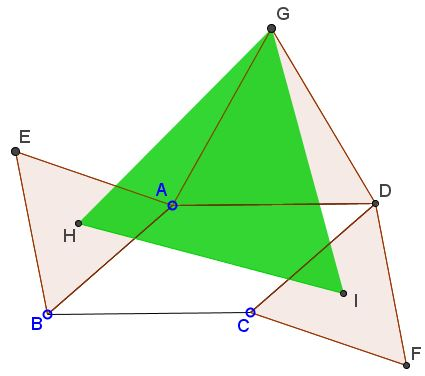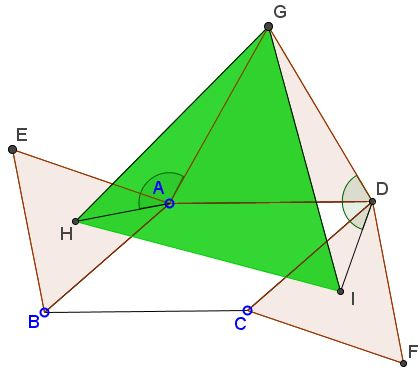# Parallelogram and Four Equilateral Triangles

### Problem

$ABCD$ a parallelogram, $ABE,$ $CDF,$ and $DGA$ are similarly oriented equilateral triangles; $H$ is the center of $\Delta ABE,$ $I$ that of $\Delta CDF.$Prove that $\Delta GHI$ is equilateral.

### Solution

Consider triangles $GAH$ and $GDI.$Certainly, $GA=GD$ and $AH=DI.$ Also, let $\angle ABC=\alpha.$ Then

\begin{align} \angle GAH &=360^{\circ}-(180^{\circ}-\alpha)-60^{\circ}-30^{\circ}=90^{\circ}+\alpha,\\ \angle GDI &=\alpha +60^{\circ}+30^{\circ}=90^{\circ}+\alpha. \end{align}

So that the two angles, and, therefore, the two triangles are equal. In particular, $\angle AGH=\angle DGI,$ implying

$\angle HGI = \angle AGD +\angle AGH -\angle DGI=\angle AGD=60^{\circ}.$

Since $GH=GI,$ $\Delta GHI$ is isosceles with the apex angle of $60^{\circ},$ so it in fact is equilateral.

### Acknowledgment

The problem has been posted by Dao Thanh Oai (Vietnam) at the Short Mathematical Idea facebook page in the name of Miguel Ochoa Sanchez (Peru).

• Angle Trisectors on Circumcircle
• Equilateral Triangles On Sides of a Parallelogram
• Pompeiu's Theorem
• Pairs of Areas in Equilateral Triangle
• The Eutrigon Theorem
• Equilateral Triangle in Equilateral Triangle
• Seven Problems in Equilateral Triangle
• Spiral Similarity Leads to Equilateral Triangle
• A Pedal Property in Equilateral Triangle
• Miguel Ochoa's van Schooten Like Theorem
• Two Conditions for a Triangle to Be Equilateral
• Incircle in Equilateral Triangle
• When Is Triangle Equilateral: Marian Dinca's Criterion
• Barycenter of Cevian Triangle
• Excircle in Equilateral Triangle
• Converse Construction in Pompeiu's Theorem
• Wonderful Trigonometry In Equilateral Triangle
• 60o Angle And Importance of Being The Other End of a Diameter
• One More Property of Equilateral Triangles
• Van Khea's Quickie
• Equilateral Triangle from Three Centroids
•